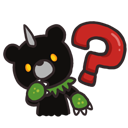# Carve Owl dmg ? Need Help for Formula

Hi everyone.
I wanted to know exactly how is calculated carve owl dommage.
I have to much information in internet and this forum. But I don’t have the answer to the question.

I found 2 formula :
(“Summon base atk” + weapon matk) X (SPR multiplier) x (“Attack SFR”)
Maybe SPR multiplier : “Base%” * (OwnerSPR/ (OwnerLevel + 1)) ////////// OR spr x 0,3%

Now I dont understand -Summon base atk what is value ? (carve owl)

• Attack SFR
• Base%

Have you got a true formula and can explain me Or if u have the answer of all question it ll be great x)
Is realy important for choise my Weapon … (Rod, 1H mace, 2H mace)
My classe : Diev, Miko, Druid.

Thks.

For dmg chars: 2HandWeapon+Trinket

Is totaly obvious but if carve owl don’t take any dmg from 2hMace ? But only spr ? Maybe Rod is best .
But thks …

Not 100% sure right now, but i think they do scale with attack (and spr)

Im not sure so is why i need formula.

Yes, they do

Summary

edit
and less SPR (ark)
https://i.imgur.com/YnfpwrB.jpg

She play meta supp Priest/Oracle/Diev .
Not realy focus on Diev “Carv Owl”.

But we can see that attack and spr changes dmg…((SPR / (level + 1)) * 100)% = summon damage formula
this is then multiplied by the summon attack modifier

it would’ve been good if we had the SPR values of @Hmmmm since only the attack values (especially of a two hand mace with a large damage variability) can’t help to do the math.

Also, no info on the attributes and arts investment of Carve Owl doesn’t really help since we’re looking for a formula, not for basic info…

Edit: Here’s the summon that’s generated by the Carve Owl skill: https://tos.neet.tv/npcs/58284

as you can see, its base summon attack SFR is 100%, so the question is where in the formula we get the multiplication of the overall value to reach the SFR listed in the skill description.

Oh, if you want to use these images

and SPR is the 4th number at ‘status’ at the addon
1503 and 1423

1 Like

ok, let’s do a quick calculation here:

((1503/451))*100% = 333.26%

The summon SFR of the Owl statue as stated by the skill description is 100.8%

Now we have 2882 matk on the first picture and a damage dealt to the monster of 15830.
The monster is a level 431 Akhlass Petal Warrior, who has got a magic defense value of 7716.

Now we’ve got to input this into the damage calculation formula to get the base damage value:
(% increase factor/100) x 2882 x min {1, log10 ((2882 / (7716 + 1))^0.8 + 1)} = 15830
(% increase factor/100) x 2882 x 0.1628 = 15830
(% increase factor/100) x 469.19 = 15830
(% increase factor/100) = 33.739

33.326 x 1.008 = 33.59

So, we can be sure that the SFR of Carve Owl is in fact the SFR of the summon as seen in the skill description, and is multiplied with the summon damage formula I listed above.
The minor deviation in the final amount can probably be explained with rounding of the game, my rounding in the formula and some other things that might influence the damage like the Ark that adds +2.1% summon damage at stage 3.

So all in all we now know the damage formula for the Carve Owl skill @mickvenle :

[ Carve Owl skill factor x ((SPR / (level + 1))] x attack x min {1, log10 ((attack / (defense + 1))^0.8 + 1)}

2 Likes

Realy THKS !! <3. You help me a lot x)
And I’m not sure x) but (level +1) = (Lvl Monster +1) ?
“Care owl skill factor” = Summon skill factor = 100,8% ? (In this example)
“Attack” = my matk total ?
“Defense” => Monster magic def.

Can we try again but this time with my character ?
My Matk : 18183
My lvl 436
My SPR :1569
My INT: 836
Carve Owl : Summon Skill factor : 326%

Enemie is : Ponta (Vienib Shelter)
Enemie LVL : 429
Enemie magi def : 10358

With this formula how dmg I do on him normaly ?
Can you detail the calculations. <3

level is the level of your character, obviously.

Here is the formula :

{Carve Owl skill factor x [SPR / (level + 1)]} x attack x min {1, log10 [((attack / (defense + 1))^0.8 + 1]}
Here it’s filled with your values, which you should recognize

{326 x [1569/(436+1)]} x 18183 x {log10 [((18183 / (10358 + 1))^0.8 + 1]} =

11,7 x 18183 x 0.41 = 87223 damage

The final value is after rounding up/down, so if it differs a little ingame that’s because the game also rounds up/down, and I’ve got no idea where and how it is rounding up/down, so 100% accurate prognosis of damage is ± impossible.
The value should, however, lie somewhere within the 5% error margin (± 4361) to still be able to be called “accurate”.

There is no real need to detail the calculations, it’s simple school math you can do with a calculator on PC in a few minutes.

Its for sur if i fail i see where x). Is not obvious for me because : more i Up lvl less i do dmg … If i do not change SPR/INT or matk. But thks !!!

legend froster lord improves the damage of owls correct?